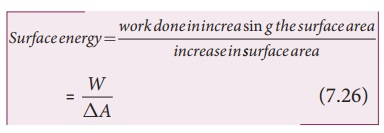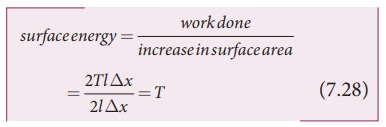Home | | Physics 11th std | Surface energy (S.E.) and surface tension (S.T.)

# Surface energy (S.E.) and surface tension (S.T.)

A molecule inside the liquid is being pulled in all direction by other molecules that surround it.

Surface energy (S.E.) and surface tension (S.T.)

## Surface Energy

Consider a sample of liquid in a container. A molecule inside the liquid is being pulled in all direction by other molecules that surround it. However, near the surface, a molecule is pulled down only by the molecules below them and there is a net downward force. As a result, the entire surface of the liquid is being pulled inward. The liquid surface thus tends to have the least surface area. To increase the surface area, some molecules are brought from the interior to the surface. For this reason, work has to be done against the forces of attraction. The amount of work done is stored as potential energy. Thus, the molecules lying on the surface possess greater potential energy than other molecules. This excess energy per unit area of the free surface of the liquid is called ŌĆśsurface energyŌĆÖ. In other words, the work done in increasing the surface area per unit area of the liquid against the surface tension force is called the surface energy of the liquid.It is expressed in JŌĆåm-2 or NŌĆåm-1 .

## Surface tension

The surface tension of a liquid is defined as the energy per unit area of the surface of a liquidThe SI unit and dimensions of T are N mŌłÆ1 and M TŌłÆ2, respectively.

## Relation between surface tension and surface energy:

Consider a rectangular frame of wire ABCD in a soap solution (Figure 7.25). Let AB be the movable wire. Suppose the frame is dipped in soap solution, soap film is formed which pulls the wire AB inward due to surface tension. Let F be the force due to surface tension, then

F = (2T)l

here, 2 is introduced because it has two free surfaces. Suppose AB is moved by a small distance Ōłåx to new a position A'B'. Since the area increases, some work has to be done against the inward force due to surface tension.

Work done = Force ├Ś distance = (2T l) (╬öx)

Increase in area of the film ŌłåA = (2l)

(╬öx)=2l  x

Therefore,Hence, the surface energy per unit area of a surface is numerically equal to the surface tension.## EXAMPLE 7.10

Let 2 .4├Ś10ŌłÆ4 J of work is done to increase the area of a film of soap bubble from 50 cm2 to 100 cm2. Calculate the value of surface tension of soap solution.

Solution:

A soap bubble has two free surfaces, therefore increase in surface area ŌłåA = A2ŌłÆA1 = 2(100-50) ├Ś 10-4m2 = 100 ├Ś 10-4m2.

Since, work done W = T ├Ś╬öA ŌćÆT =Study Material, Lecturing Notes, Assignment, Reference, Wiki description explanation, brief detail
11th Physics : UNIT 7 : Properties of Matter : Surface energy (S.E.) and surface tension (S.T.) |